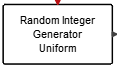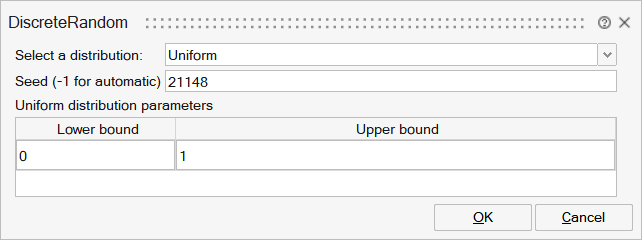# DiscreteRandom

This block is a random signal generator based on discrete probability distribution. Each output component takes piecewise constant random values. Every time an event is received on the input event port, the outputs take new independent random values. Output port size is given by the size of the parameters matrices. You can choose the distribution.SignalGenerators

## Description

The DiscreteRandom block is a discrete random wave generator. Each output component is an integer value (int32) and takes piecewise constant random values. Every time an event is received on the activation port, the outputs take new independent random values. Output port size is given by the size of the parameters matrices.

Supported distributions are: Bernoulli, Binomial, Poisson, Uniform, Geometric, HyperGeometric, Logarithminc, Negative Binomial.

## ParametersNameLabelDescriptionData TypeValid Values

distrib

Select a distribution:

Probability distribution. Default value: 'uniform'

Structure

distrib/bernoulli

Bernoulli

Number

0
1

distrib/binomial

Binomial

Number

0
1

distrib/poisson

Poisson

Number

0
1

distrib/uniform

Uniform

Number

0
1

distrib/geometric

Geometric

Number

0
1

distrib/hgeometric

Hypergeometric

Number

0
1

distrib/log

Logarithmic

Number

0
1

distrib/neg_binomial

Negative binomial

Number

0
1

seed

Seed (-1 for automatic)

Seed for the random generator (scalar). seed must be an integer between 0 and 2^32-1. Negative value for seed means automatic selection.

Matrix

Bern

Bernoulli distribution parameters

Bernoulli probability distribution parameters.

Structure

Bern/p

Success probability (0&lt;p&lt;1)

Cell of matrices

Bino

Binomial parameters

Binomial probability distribution parameters.

Structure

Bino/N

Number of trials (&gt;0)

Cell of matrices

Bino/P

Probability of success

Cell of matrices

Pois

Poisson distribution parameters

Poisson probability distribution parameters.

Structure

Pois/lambda

Lambda (&gt;0)

Cell of matrices

Unif

Uniform distribution parameters

Uniform probability distribution parameters.

Structure

Unif/A

Lower bound

Cell of matrices

Unif/B

Upper bound

Cell of matrices

Geo

Geometric distribution parameters

Geometric probability distribution parameters.

Structure

Geo/p

success probability (0&lt;p&lt;1)

Cell of matrices

HGeo

HyperGeometric distribution parameters

Hypergeometric probability distribution parameters.

Structure

HGeo/N

Population size

Cell of matrices

HGeo/m

Number of tagged items

Cell of matrices

HGeo/nn

Number of draws

Cell of matrices

Log

Logarithmic distribution parameters

Logarithmic probability distribution parameters.

Structure

Log/theta

Probability

Cell of matrices

NBino

Negative binomial distribution parameters

Negative binomial probability distribution parameters.

Structure

NBino/r

Number of successes (&gt;0)

Cell of matrices

NBino/p

probability of a success (0&lt;p&lt;1)

Cell of matrices

## Ports

NameTypeDescriptionIO TypeNumber

Port 1

explicit

output

1

Port 2

activation

input

1

NameValueDescription

always active

no

Time Dependency = standard mode activated

direct-feedthrough

no

zero-crossing

no

mode

no

continuous-time state

no

discrete-time state

yes